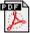CSML 2007 reading group on Stochastic Differential Equations

Overview

We are interested in the modelling of continuous-time continuous state stochastic processes from uncertain observations. Such processes, also known as diffusion processes, describe dynamical systems andarise in a wide range of applications, e.g. numerical weather prediction, finance and genetic networks. Often, we may assume that the dynamical models are formulated by systems of differential equations.

The reading group will first study the theory of diffusion processes and their mathematical description by stochastic differential equations (SDEs). We are interested in the basics of Ito stochastic calculus, solvable SDEs, stochastic Taylor expansions and time discrete approximation of SDEs.

Next, recent advances in the field will be discussed. To mention one example, when following a Bayesian approach, we may incorporate a priori knowledge about the dynamics by providing probability distributions on the unknown functions, which correspond for example to driving forces and appear as coefficients or parameters in the differential equations. Hence, such functions become stochastic processes in a probabilistic Bayesian framework.

The main reference material for the reading group (RG) can be found in Numerical Solutions to Stochastic Differential Equations by Kloeden and Platen (1999, second edition). The reading groups will take place on Thursdays from 11:00 until 12:30, usually in room 6.12 or 6.12a, Malet Place Engineering Building, Department of Computer Science, University College London.

 18/01 (room 6.12) A Short Introduction to Diffusion Processes and Ito Calculus C. Archambeau (CSML, UCL) The first RG will cover some basic concepts on measure and probability theory, the basic properties of stochastic processes, Markov processes and Diffusion processes. Finally, we will discuss Ito stochastic calculus, i.e. the Ito (stochastic) integral and the Ito formula (stochastic chain rule). Presentation& notes. 25/01 (room 6.12) Modelling with Stochastic Differential Equations C. Archambeau (CSML, UCL) and Y. Shen (NCRG, Aston University) During the second RG, we will first discuss general solutions to linear SDEs and reducible SDEs. Next, we will briefly discuss the difference between Ito and Stratonovich stochastic calculus, as well as several modelling schemes (parameter estimation, optimal stochastic control and filtering). Presentation part I& part II. 08/02 (room 6.12a) An Introduction to Using SDEs Cancelled!! Lee Harrison (FIL, UCL) In this RG, we will cover the Ito-Taylor expansion, Euler/Milstein discretization schemes, strong/weak convergence and stability. 15/02 (FIL seminar) An Introduction to Using SDEs Lee Harrison (FIL, UCL) In this RG, we will cover the Ito-Taylor expansion, Euler/Milstein discretization schemes, strong/weak convergence and stability. Presentation. 19/04 (room 6.12a) Discussion Paper: Nonlinear EEG Analysis based on a Neural Mass Model by Valdes et al. Lee Harrison(FIL, UCL)P.A. Valdes, J.C. Jimenez, J. Riera, R. Biscay, T. Ozak, Nonlinear EEG Analysis based on a Neural Mass Model, Biological Cybernetics 81, 415-424 (1999). Presentation. 03/05 (room 6.12) Discussion Paper: Gaussian Process Approximations of Stochastic Differential Equations C. Archambeau (CSML, UCL)C. Archambeau, D. Cornford, M. Opper, J. Shawe-Taylor, Gaussian Process Approximations of Stochastic Differential Equations, JMLR Workshop and Conference Proceedings, 1:1-16, 2007. Presentation.